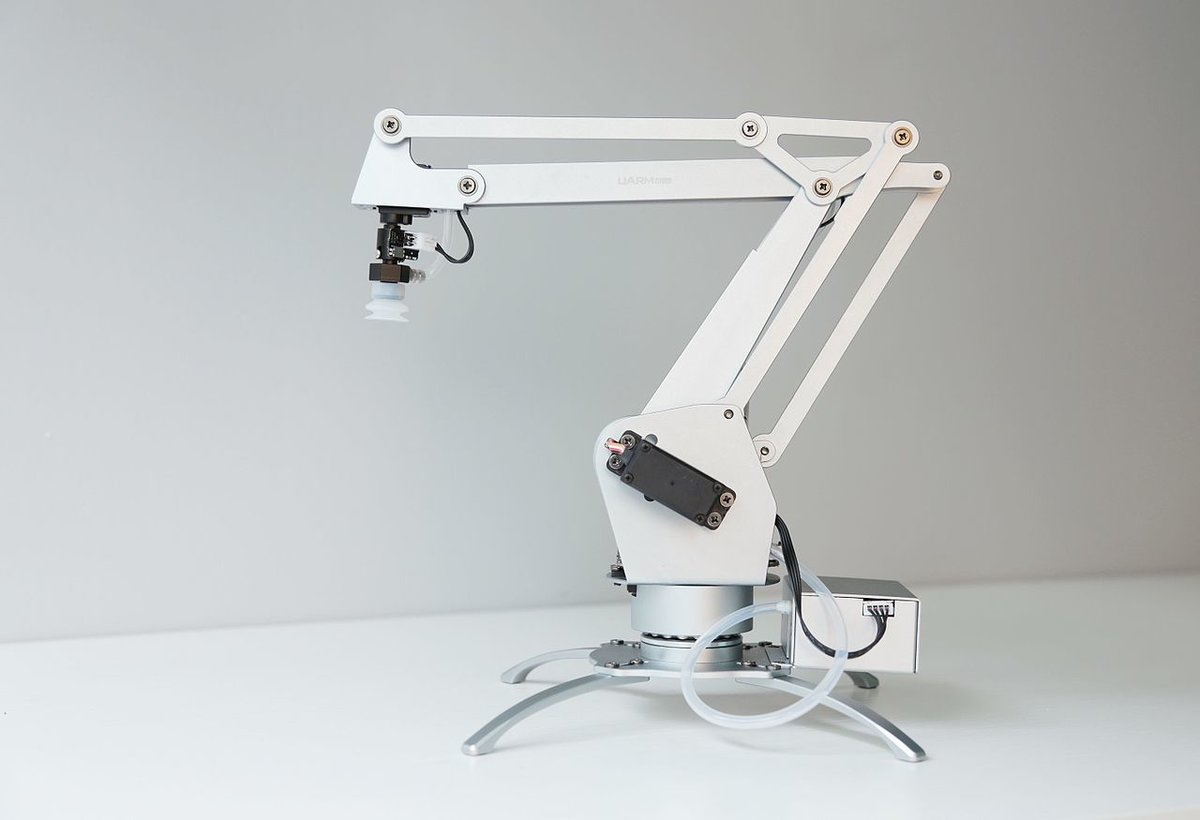# Difference Between Forward Kinematics and Inverse KinematicsIn previous tutorials, we used known joint variables (i.e. servo motor angles, displacement of a linear actuator, etc.) to calculate the position and orientation of the end effector of a robotic arm (e.g. robotic gripper, robotic hand, vacuum suction cup, etc.) in 3D space. This is called forward kinematics.

Forward kinematics asks the question: Where is the end effector of a robot (e.g. gripper, hand, vacuum suction cup, etc.) located in space given that we know the angles of the servo motors?

Inverse kinematics is the forward kinematics problem in reverse. We know the position and orientation we want the end effector of a robotic arm to have, and we want to find the values of the joint variables that generate that desired position and orientation of the end effector.

• Joint Variables —–> Pose of the End effector of a Robotic Arm = Forward Kinematics
• Pose of the End effector of a Robotic Arm —–> Joint Variables = Inverse Kinematics

Inverse kinematics asks the question: What do the angles of the servo motors need to be given our desired position and orientation of the end effector of a robotic arm (e.g. gripper, hand, vacuum suction cup, etc.)?

Cover Image Source: Wikipedia.